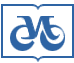Publishing House SB RAS:

Address of the Publishing House SB RAS:
Morskoy pr. 2, 630090 Novosibirsk, Russia

Home – Home – Jornals – Journal of Structural Chemistry 2009 number 6

Journal of Structural Chemistry

2009 year, number 6

RELATIONSHIP BETWEEN THE BOND LENGTHS IN N-H…N, O-H…O, F-H…F, AND Cl-H…Cl HYDROGEN BRIDGES

E. G. Tarakanova, G. V. Yukhnevich
Keywords: hydrogen bridge, H-bond length, quantum-chemical calculation
Pages: 1063-1069

Abstract

The applicability of the equation e-((r1-r0)/b)5/3 + e-((r2-r0)/b)5/3 = 1 has been studied. The equation defines the relationship between the experimental values of the covalent (r1) and hydrogen (r2) bond lengths in O-H…O bridges for describing the relation between the experimental interatomic distances in N-H…N bridges and the parameters of X-H…X fragments (X = O, N, F, Cl) calculated by the density functional method (B3LYP/6-31++G(d,p)) for neutral, positive, and negative molecular complexes. Here r0 is the mean value of the X-H bond length in free molecules; rsym is the X…H distance in the symmetrical bridge; and b is the coefficient defined by the equation b = (rsym - r0)/(ln2)3/5. This equation allows us to adequately describe the relationships between bond lengths in nearly linear hydrogen bridges formed by oxygen, nitrogen, fluorine, and chlorine atoms. It is thus universal and can be used in studies of a wide range of substances.